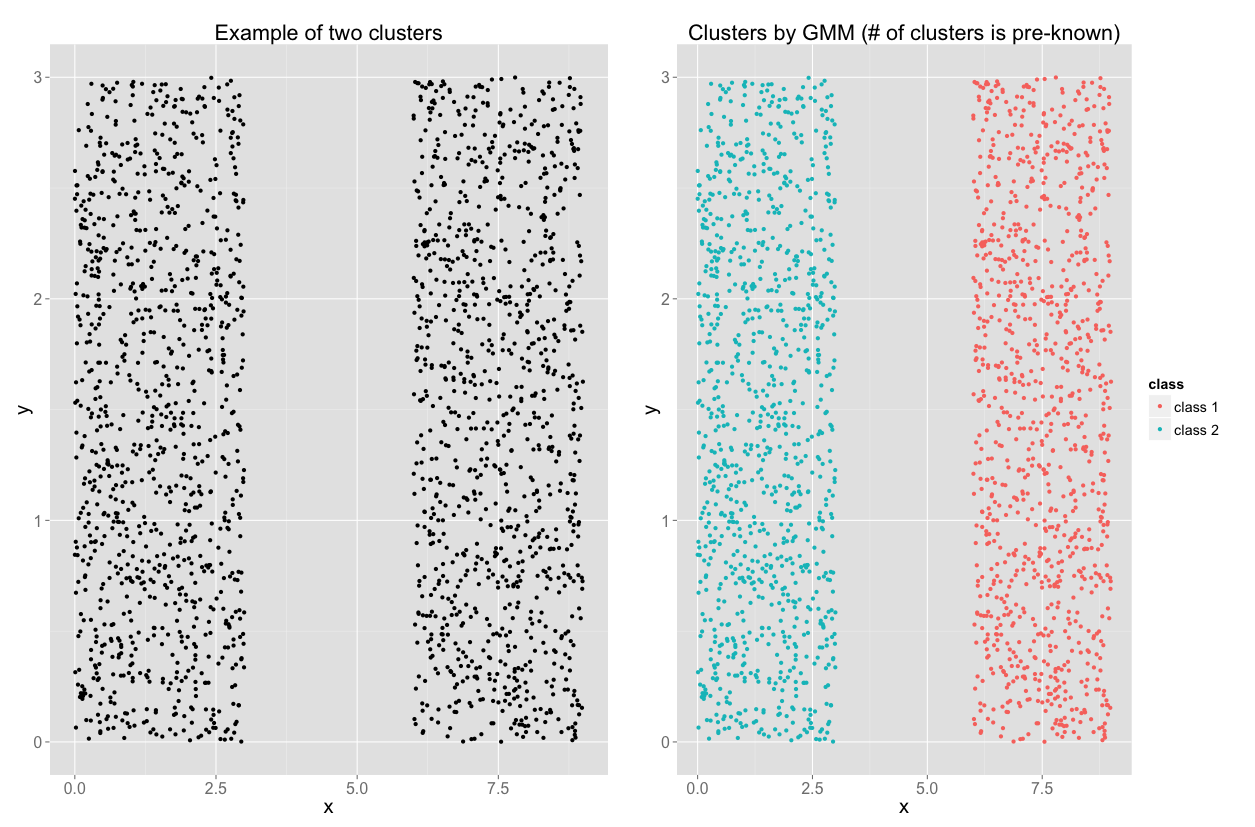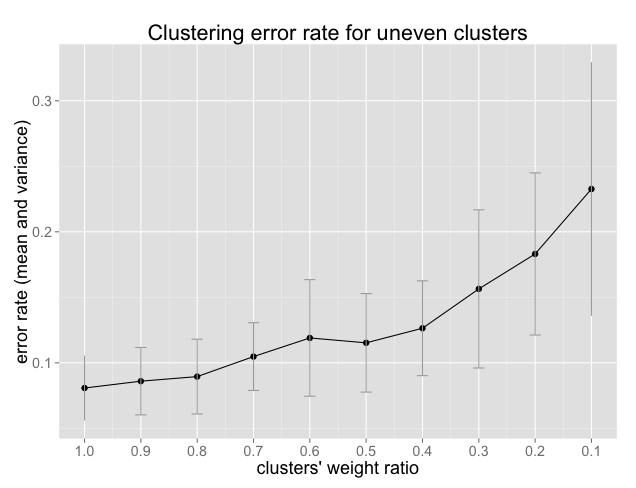When not to use Gaussian Mixture Model (EM clustering)

An universally used generative unsupervised clustering is Gaussains Mixture Model (GMM) which is also known as "EM Clustering". The idea of GMM is very simple: for a given dataset, each point is generated by linearly combining multiple multivariate Gaussians:

Eq. (1)

In other words, the idea of the EM clustering is that there are K clusters and points in j-th cluster are following a normal distribution with mean μand covariance matrix Σj. Each point xi in the dataset has a soft assignment to the K clusters. This soft assignment is determined by πj N(xi|μj, Σj). One can convert this soft probabilistic assignment into membership by picking up the most likely clusters (cluster with highest probability of assignment).

Similar to other clustering algorithm, the GMM has some assumptions about the format and shape of the data. If those criteria is not meet the performance might drop significantly. The point of this post is to investigate the performance of EM clustering in the following scenarios:
• Non-Gaussian dataset: as it is clear from the formulation, GMM assumes an underlying Gaussian generative distribution. However, many practical datasets do not satisfy this assumption. I study effect of non-Gaussian dataset in two cases:
• The number of clusters is known
• The number of clusters is unknown
• Uneven cluster sizes. When clusters do not have even sizes there is a high chance that small cluster gets dominated by the large one.
For this post I am using the R EMCluster package.

Example:
Let start with an example. Consider the dataset depicted on the left side of the following figure. There are two clusters in this dataset and clearly neither of them are following normal distribution. However, if we feed this to the EM clustering with K=2 (assuming number of cluster is known), it will recognize the two clusters and generate the result on the right hand side.Fig. 1: Example of GMM clustering
This is great. Does this mean that the Gaussian assumption is just for formulation and EM clustering is doing a good job even if the data is not normal.

The answer is: it depends on the dataset and the information you give to the clustering routine.

Let study this more.

1- Non-Gaussian dataset
A. Number of clusters is known
When the data is not normal, there is no guarantee that EM clustering will pickup the right clusters. Look at the following example. Clearly, the data on the left has two clusters. However, the GMM clustering can not recognize the two.

(In case you are wondering if there is any clustering algorithm that can do a good job in this dataset, you might give the hierarchical clustering, hclust in R, a try.)Fig.2: If data is not Gaussian, GMM clustering could not recognize right clusters
B. Number of clusters is unknown
As it is seen in Eq. (1), EM clustering requires to know the number of clusters (K) in advance. But what if you do not know the number of clusters? what if you want to use EM clustering in the production and number of clusters are different for different customers?

Similar to other clustering approaches, one can use an Information Criteria, such as Bayesian Information Criterion (BIC) or Akaike information criterion (AIC), to find the best K. Here I use BIC method. EMCluster package has em.bic function that gets an EM object and calculate its BIC.

If the clusters in the dataset have normal distribution, then BIC method easily finds the optimum (and usually) correct number of clusters. The example is given below.Fig. 3: If data is Gaussian, we can find number of clusters using BIC

But if the data is not normal then, BIC will be faulty in terms of correct number of clusters. Let use the data set in Figure 1. This time instead of telling the EM clustering that K=2, we try to find the best K. Well the result is not promising. The BIC suggest to have 12 clusters. Why? Because those 12 clusters will have normal distribution that matches the underlying assumption of GMM.Fig 4: If data is not Gaussian, correct number of clusters is hard to find. Because GMM finds number of clusters that all have Normal distribution.

2- Uneven clusters
What if the clusters have an uneven number of points. Ideally the πj in Eq. (1) will take care of uneven cluster size. In other words, clusters can have different weights. However, due to the sub optimality of EM algorithm, if there is large difference between the size of the clusters then error rate might be significant. To prove this point, let's create two Gaussian clusters where size of one is α times of the other one ( 0 < α <1). The we run the EM clustering and calculate the error rate (rate of miss classification). The following plot shows the error rate for each value of α. As one can see, the more uneven cluster sizes are, the higher the error rate is. Moreover, the variance of error rate gets wider for lower value of α (lower value of α means one cluster has less number of points than the other one).Fig 5: Uneven cluster size leads to higher misclassification error rate

1.2D GMMs are "soft" ovals. If you can use ovals to surround your clusters, GMM will do a good job - hence the first figure, you could surround each of the rectangular clusters with an oval. On the other hand, for the second figure, you cannot surround the inside cluster and the outside cluster with separate ovals. hclust is a great algorithm, spectral and graph-based clustering algorithms could also tackle such cases rather well.

1.Thanks Amir, good point about ovals. However, note that for non-ellipsis ovals, GMM does a good job If you know the number of clusters in advance. In Fig 4, I used BIC method to find the best number of clusters. As you can see, 12 clusters was the optimum answer.

2.I was wondering, when our data is non Gaussian, which algorithm give the better cluster? Since GMM gives bias result.
thank you.

Favorite Quotes

"I have never thought of writing for reputation and honor. What I have in my heart must out; that is the reason why I compose." --Beethoven

"All models are wrong, but some are useful." --George Box